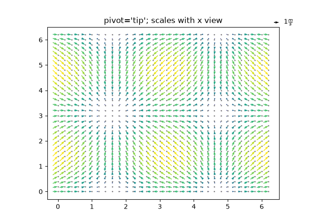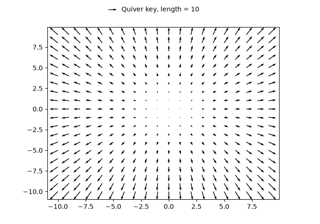# matplotlib.axes.Axes.quiverkey#

Axes.quiverkey(Q, X, Y, U, label, **kwargs)[source]#

Add a key to a quiver plot.

The positioning of the key depends on X, Y, coordinates, and labelpos. If labelpos is 'N' or 'S', X, Y give the position of the middle of the key arrow. If labelpos is 'E', X, Y positions the head, and if labelpos is 'W', X, Y positions the tail; in either of these two cases, X, Y is somewhere in the middle of the arrow+label key object.

Parameters:
Q`matplotlib.quiver.Quiver`

A `Quiver` object as returned by a call to `quiver()`.

X, Yfloat

The location of the key.

Ufloat

The length of the key.

labelstr

The key label (e.g., length and units of the key).

anglefloat, default: 0

The angle of the key arrow, in degrees anti-clockwise from the x-axis.

coordinates{'axes', 'figure', 'data', 'inches'}, default: 'axes'

Coordinate system and units for X, Y: 'axes' and 'figure' are normalized coordinate systems with (0, 0) in the lower left and (1, 1) in the upper right; 'data' are the axes data coordinates (used for the locations of the vectors in the quiver plot itself); 'inches' is position in the figure in inches, with (0, 0) at the lower left corner.

colorcolor

Overrides face and edge colors from Q.

labelpos{'N', 'S', 'E', 'W'}

Position the label above, below, to the right, to the left of the arrow, respectively.

labelsepfloat, default: 0.1

Distance in inches between the arrow and the label.

labelcolorcolor, default: `rcParams["text.color"]` (default: `'black'`)

Label color.

fontpropertiesdict, optional

A dictionary with keyword arguments accepted by the `FontProperties` initializer: family, style, variant, size, weight.

**kwargs

Any additional keyword arguments are used to override vector properties taken from Q.

## Examples using `matplotlib.axes.Axes.quiverkey`#